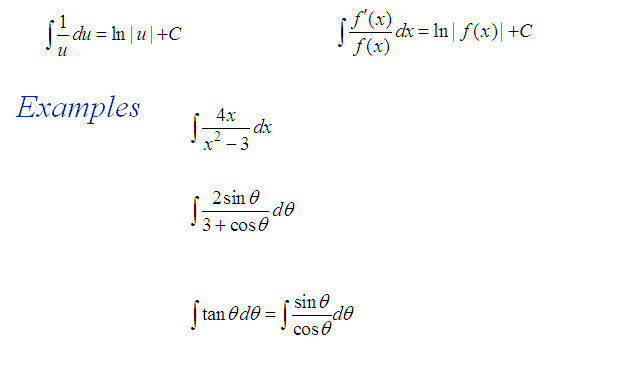# SCC Education

## Integration ,derivatives involving the natural log function,exponential function, derivative of inverse sine,derivatives of inverse trig function,

Integration /derivatives involving the natural log functionsome-important-formulas-of-integration

solving-models-related-to-ordinary differential equation

differentiation-of-exponential-functions

area-between-curve

vector

application-of-derivatives

homogenous-differential-equation

inverse-trigonometry-questions

integration

integration-questions

Rolles-theorem-and-mean-value-theorem

Techniques-of-integration-substitution

critical-points-and-point-of-inflection

intermediate-value-theorem

### Sample paper Mathematics 12